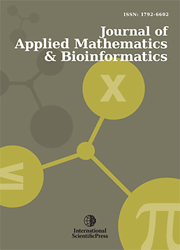# Journal of Applied Mathematics & Bioinformatics

### Aims and Scope

Journal of Applied Mathematics and Bioinformatics is a refereed journal devoted to the publication of original research papers and review articles in all areas of applied, computational, and industrial mathematics. The journal, also is concerned with high-level mathematical investigations of modern bioinformatics problems and reports current progress in this field. It is published with both online and print versions.

The journal covers the frontier issues in the applied mathematics and their applications in business, industry, science and other subjects. Applied Mathematics is a branch of mathematics that concerns itself with the connections between mathematics and other domains with the applications of the mathematical knowledge. A broad spectrum of applied mathematics is covered by the journal. The subjects include differential equations (ODEs and PDEs), numerical analysis, scientific computing, approximation theory and representation theory, matrix theory, mathematical physics, mathematical methods of engineering, optimization, operations research, linear and nonlinear programming, continuous modelling control theory, mathematical biology, bioinformatics, information theory, game theory, probability, statistics, theoretical computer science, stochastic systems theory, neuroscience, mechanics of solids, materials science and fluids etc.

`Google Scholar Citations | Google Scholar Metrics`

#### Current Issue : Volume 12, Issue 1 (2022)ISSN: 1792-6939 (Online)
1792-6602 (Print)